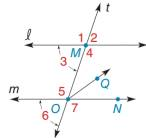Chapter 2.1, Problem 13E### Elementary Geometry for College St...

6th Edition
Daniel C. Alexander + 1 other
ISBN: 9781285195698

#### Solutions

Chapter
Section### Elementary Geometry for College St...

6th Edition
Daniel C. Alexander + 1 other
ISBN: 9781285195698
Textbook Problem
4 views

# l ∥ m , with transversal t and O Q → bisects ∠ M N O . If m ∠ 1 = 112 ° , find the following: a) m ∠ 2 b) m ∠ 4 c) m ∠ 5 d) m ∠ M O QExercise 15, 16

To determine

a)

To find:

The measure of the given angle.

Explanation

The sum of angles that falls on the straight line is 180°.

Given:

The given figure is,

lm, with transversal t, OQ bisects MNO and m1=112°.

The given angle is 2.

Calculation:

Consider the given figure,

From the figure, lm with transversal t.

Angles, 1 and 2 fall on the straight line l

To determine

b)

To find:

The measure of the given angle.

To determine

c)

To find:

The measure of the given angle.

To determine

d)

To find:

The measure of the given angle.

### Still sussing out bartleby?

Check out a sample textbook solution.

See a sample solution

#### The Solution to Your Study Problems

Bartleby provides explanations to thousands of textbook problems written by our experts, many with advanced degrees!

Get Started

#### In Exercises 13-20, sketch a set of coordinate axes and plot each point. 15. (3, 1)

Applied Calculus for the Managerial, Life, and Social Sciences: A Brief Approach

#### Show that 0x2ex2dx=120ex2dx.

Single Variable Calculus: Early Transcendentals

#### Find if , y = sin 2t.

Study Guide for Stewart's Multivariable Calculus, 8th

#### True or False: converges.

Study Guide for Stewart's Single Variable Calculus: Early Transcendentals, 8th